# A Y = A ® B 1 1 1 1 1 Apply the idea of the truth table above and design a 3-bit comparator using XOR or XNOR gates of...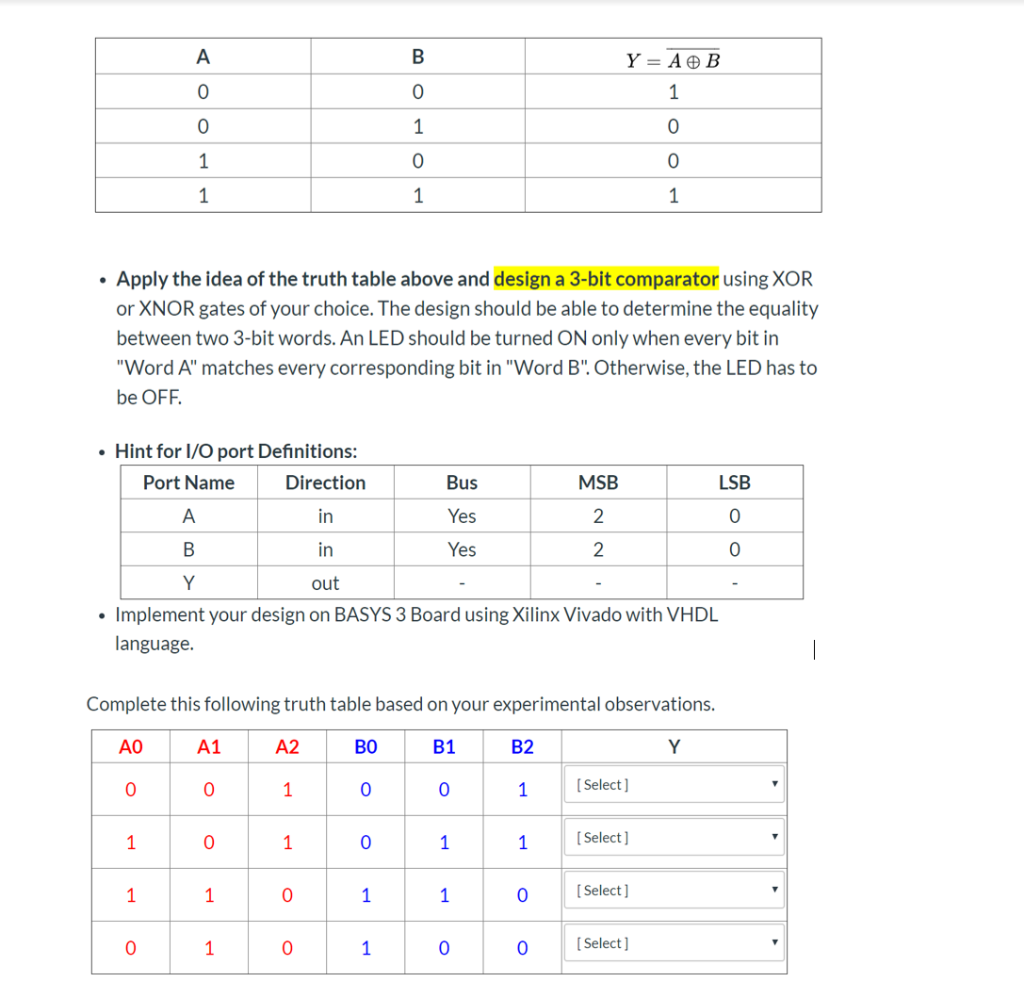A Y = A ® B 1 1 1 1 1 Apply the idea of the truth table above and design a 3-bit comparator using XOR or XNOR gates of your choice. The design should be able to determine the equality between two 3-bit words. An LED should be turned ON only when every bit in "Word A" matches every corresponding bit in "Word B". Otherwise, the LED has to be OFF Hint for I/O port Definitions: Port Name Direction Bus MSB LSB A in Yes 2 Yes in 2 Y out Implement your design on BASYS 3 Board using Xilinx Vivado with VHDL language Complete this following truth table based on your experimental observations. AO A1 A2 BO B1 B2 Y [ Select ] 1 1 Select 1 1 0 1 1 [Select] 1 1 0 1 1 [Select] 1 0 C

VHDL Code for the 3 bit equality comparator is below:

-- In the 1 bit exnor gate, when the two inputs A abd B are equal then we get the Y = '1';
-- so we will use this feature of exnor gate to form a 3 bit comparator

library ieee;
use ieee.std_logic_1164.all;
use ieee.std_logic_arith.all;

entity comparator is
port(
A : in std_logic_vector (2 downto 0);
B : in std_logic_vector (2 downto 0);
Y : out std_logic
);
end comparator;

architecture behave of comparator is
signal eq0, eq1, eq2 : std_logic;
begin

eq2 <= not (A(2) xor B(2));
eq1 <= not (A(1) xor B(1));
eq0 <= not (A(0) xor B(0));
-- all of the bits are equal then only Y = '1' else not
Y <= eq0 and eq1 and eq2;

end behave;

VHDL Testbench code for the above design code is:

library ieee;
use ieee.std_logic_1164.all;

entity comparator_tb is
end comparator_tb;

architecture behavior of comparator_tb is

component comparator
port(
A : in std_logic_vector(2 downto 0);
B : in std_logic_vector(2 downto 0);
Y : out std_logic
);
end component;

--Inputs
signal A : std_logic_vector(2 downto 0) := (others => '0');
signal B : std_logic_vector(2 downto 0) := (others => '0');

--Outputs
signal Y : std_logic;

begin

-- Instantiate the Unit Under Test (UUT)
uut: comparator port map (
A => A,
B => B,
Y => Y
);

-- Stimulus process
stim_proc: process
begin
A <= "001"; B <= "001"; wait for 50 ns;
A <= "101"; B <= "011"; wait for 50 ns;
A <= "110"; B <= "110"; wait for 50 ns;
A <= "010"; B <= "100"; wait for 50 ns;
wait;
end process;

end;

Wave form for the output :-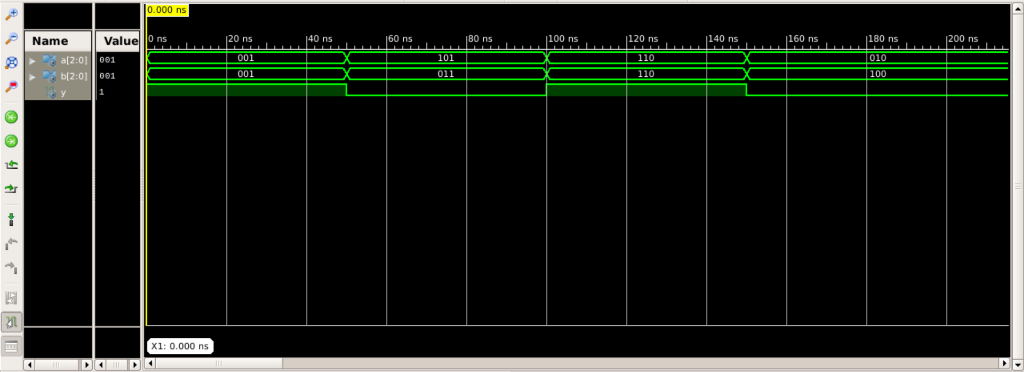##### Add Answer of: A Y = A ® B 1 1 1 1 1 Apply the idea of the truth table above and design a 3-bit comparator using XOR or XNOR gates of...
Similar Homework Help Questions
• ### A Y = A+ B 1 1 1 1 1 1 Apply the idea of the truth table above and design a 3-bit comparator using XOR or XNOR gates of...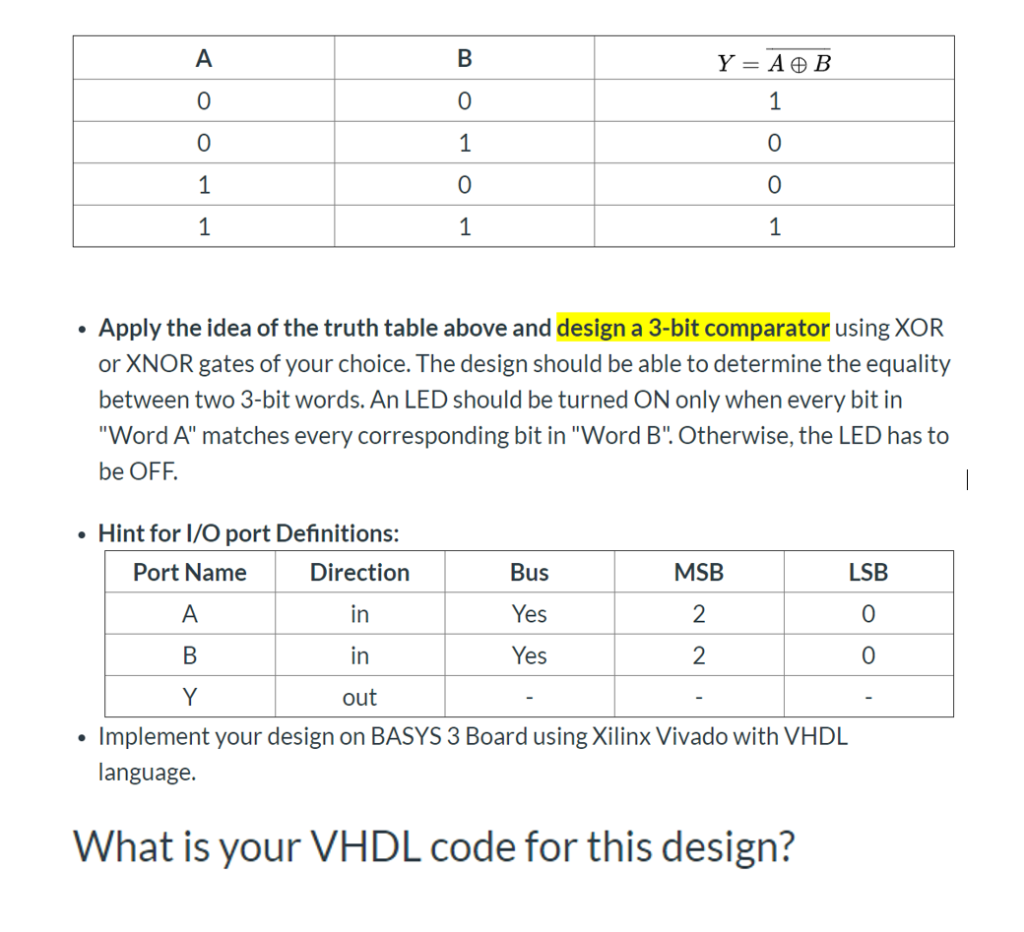A Y = A+ B 1 1 1 1 1 1 Apply the idea of the truth table above and design a 3-bit comparator using XOR or XNOR gates of your choice. The design should be able to determine the equality between two 3-bit words. An LED should be turned ON only when every bit in "Word A" matches every corresponding bit in "Word B". Otherwise, the LED has to be OFF Hint for I/O port Definitions: Port Name MSB...

• ### A Y = A ® B 0 1 1 1 0 1 1 1 Apply the idea of the truth table above and design a 3-bit comparator using XOR or XNOR gat...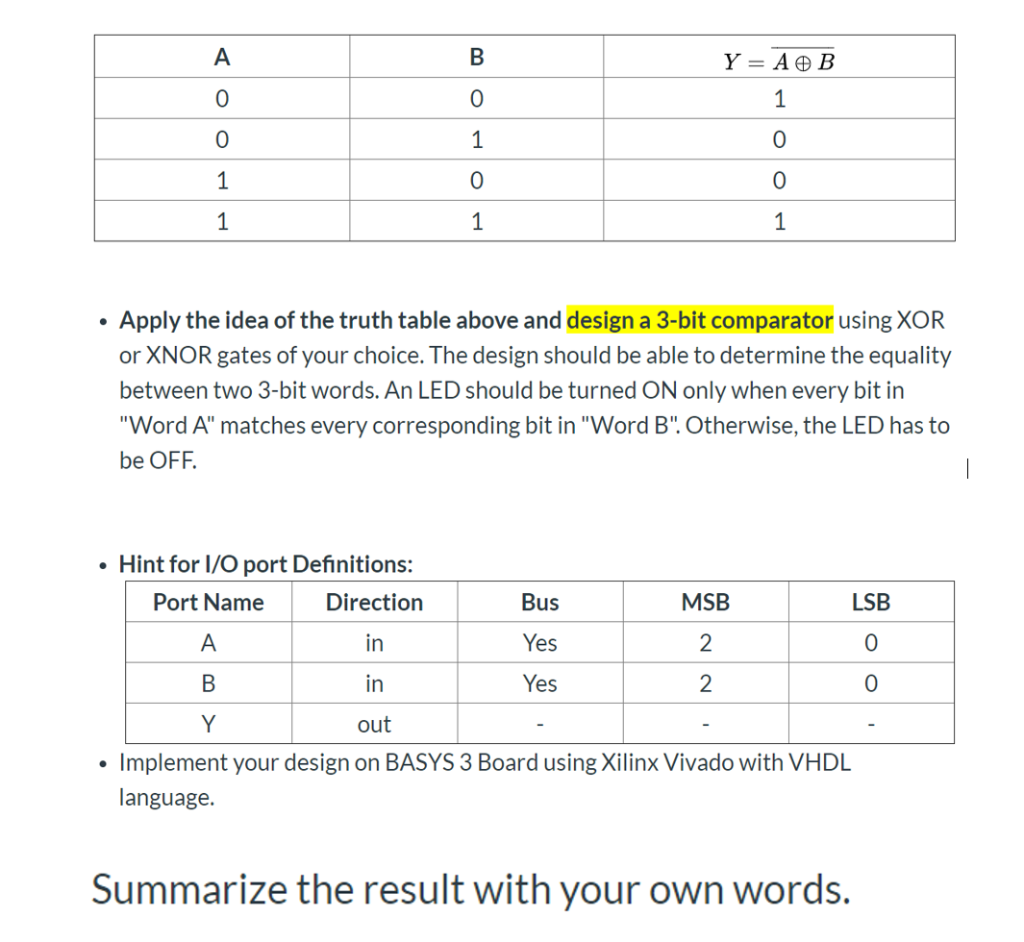A Y = A ® B 0 1 1 1 0 1 1 1 Apply the idea of the truth table above and design a 3-bit comparator using XOR or XNOR gates of your choice. The design should be able to determine the equality between two 3-bit words. An LED should be turned ON only when every bit in "Word A" matches every corresponding bit in "Word B".Otherwise, the LED has to be OFF Hint for I/O port Definitions: MSB...

• ### A Y = A ® B 1 1 1 0 0 1 1 1 Apply the idea of the truth table above and design a 3-bit comparator using XOR or XNOR gat...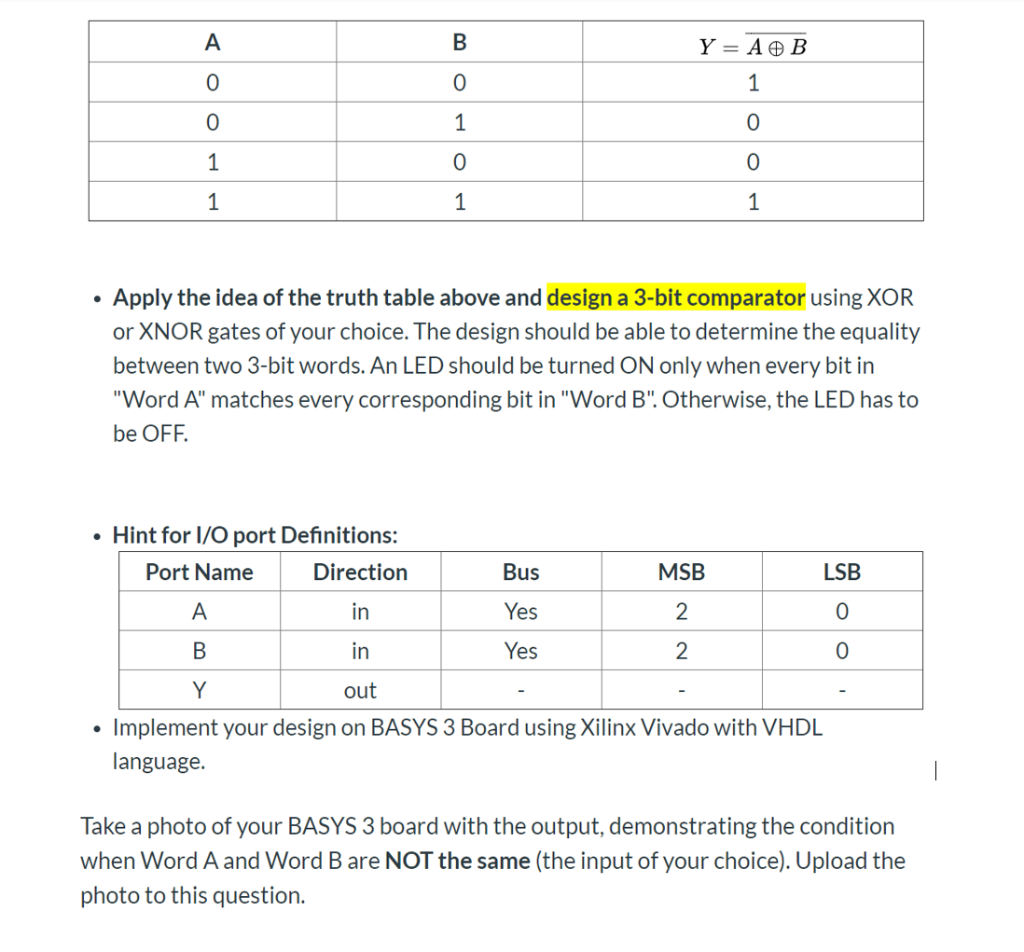A Y = A ® B 1 1 1 0 0 1 1 1 Apply the idea of the truth table above and design a 3-bit comparator using XOR or XNOR gates of your choice. The design should be able to determine the equality between two 3-bit words. An LED should be turned ON only when every bit in "Word A" matches every corresponding bit in "Word B". Otherwise, the LED has to be OFF. Hint for I/O port Definitions:...

• ### Use as few 3-input NOR gates as possible to design a bubble detector circuit for 8-bit thermomete...

Use as few 3-input NOR gates as possible to design a bubble detector circuit for 8-bit thermometer code. An n-bit thermometer code represents an integer m, with m 1s followed by (n-m) 0s. 1-bit bubble is an error in coding when a solitary 0 (or 1) is found in between two 1s (or 0s). What is the size of your circuit in terms of the number of NOR gates used? Give a gate level schematic diagram for your circuit. Implement...

• ### Design a 4-bit ALU with the truth table above. In this design A and B are two 4-bit binary inputs, s0, s1, s2, s3 and Ci...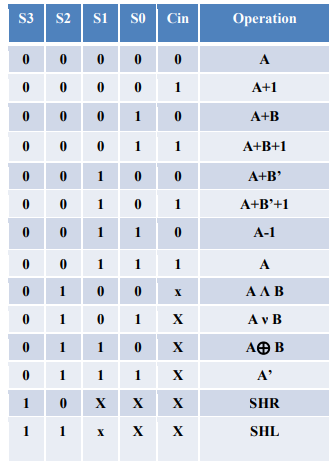Design a 4-bit ALU with the truth table above. In this design A and B are two 4-bit binary inputs, s0, s1, s2, s3 and Cin are control signals. There is no need to draw the internal circuits of MUX & Full adders but I need the logic gates drawn out. S3 S2 s1 Cin Operation 0 A 0 0 0 1 A+1 0 0 1 10 A+B 0 1 1 A+B+1 A+B 0 0 0 0 0 A+B'+1 0...

• ### Finite state machine (FSM) counter design: Gray codes have a useful property in that consecutive numbers differ in only a single bit position. Table 1 lists a 3-bit modulo 8 Gray code representing the...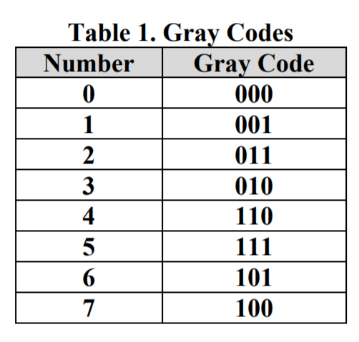Finite state machine (FSM) counter design: Gray codes have a useful property in that consecutive numbers differ in only a single bit position. Table 1 lists a 3-bit modulo 8 Gray code representing the numbers 0 to 7. Design a 3-bit modulo 8 Gray code counter FSM. a) First design and sketch a 3-bit modulo 8 Gray code counter FSM with no inputs and three outputs, the 3-bit signal Q2:0. (A modulo N counter counts from 0 to N −...

• ### 1. a. Design and implement a combinational circuit with three inputs w, x, and y and three outputs A, B and C using CMOS transistors. When the binary input is 0, 1, 2 or 3 the binary output is three g...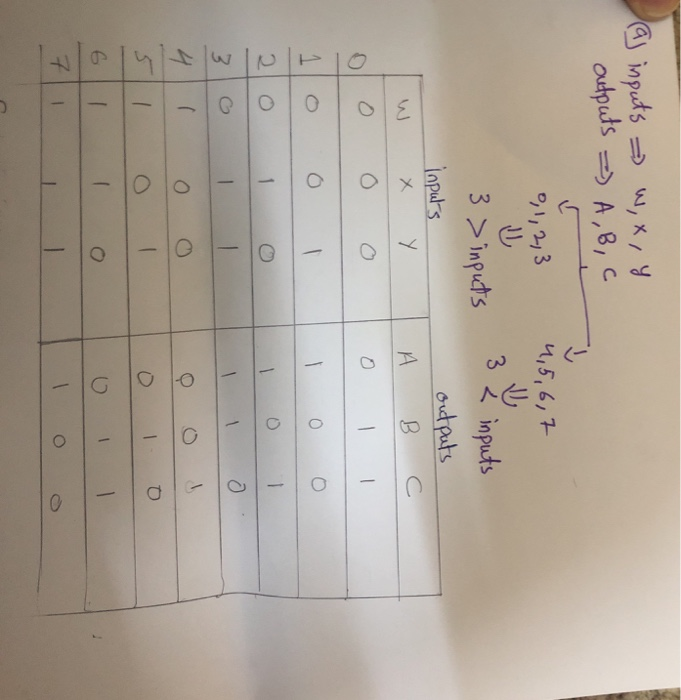1. a. Design and implement a combinational circuit with three inputs w, x, and y and three outputs A, B and C using CMOS transistors. When the binary input is 0, 1, 2 or 3 the binary output is three greater than the input. When the binary input is 4, 5, 6 or 7 the binary output is three less than the input. b. from the part (a) , Draw the mask layout with Ln = Lp= 0.6 μm, Wn=...

Need Online Homework Help?